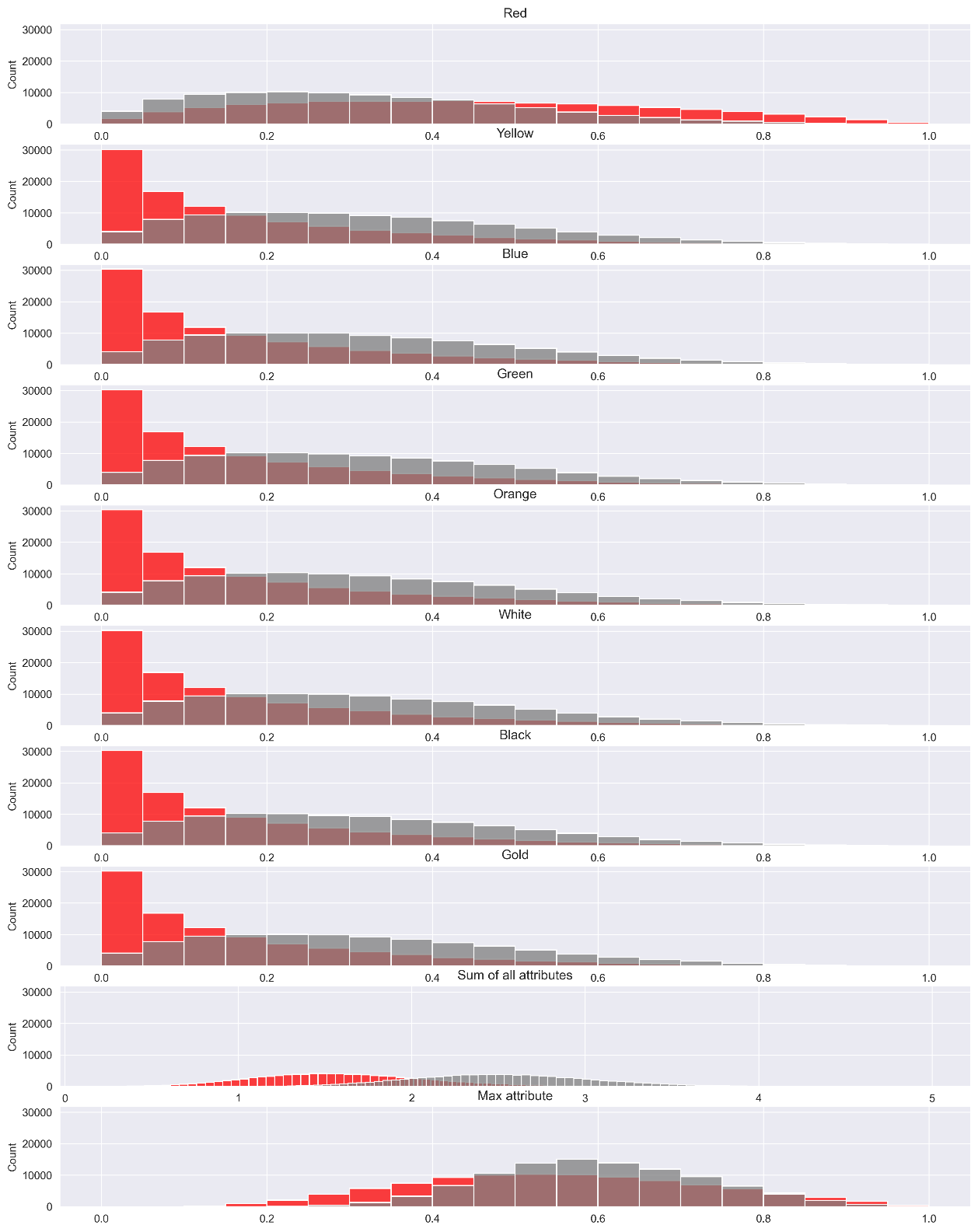# Minting (Crafting Weapons)Stalwart Mintzogi smelting blocks to mint fresh green candle swords.
MBLK are used directly in-game to mint all weapons, armor, skins and items within the game itself. Within Legends of Bezogia there are 8 different colored blocks, each representing different attributes:Colored blocks only exist in-game and are not on-chain, however, they are directly pegged to MBLK by a fixed ratio. The ratio represents the different value of each color of block based on the attribute it represents. The exact exchange rates between MBLK and in-game blocks is yet to be confirmed and will be tested and optimized during the beta phase of game testing on testnet.

### Minting Mechanics

When minting an item in the game, the total cost in blocks can be spent in any proportion of the 8 colors of blocks.
Each type of block will have a different effect on the object and affect the overall stats of the Bezogi that holds it. The main impact comes from the attribute with the highest value but others have a smaller effect on the stats of the item.
The attributes of an objects are as follows:
$\Large A=\left(a_{r e d}, a_{y e l l o w} \ldots\right)$
Where
$a_i∈[0,1]$
is the value of the attribute for the color
$i$
​.
For each color
$i$
, the attributes are computed as a from the proportion of blocks used
$b_i$
from three independent uniformly distributed random variables
$r_{1,i} , r_{2,i}, r_{3,i} \in [0,1]$
as follows:
$\Large a_{i}=\left[r_{1, i}^{v_{1}} \cdot b_{i}^{v_{2}}+r_{1, i} \cdot r_{2, i} \cdot\left(1-b_{i}^{v_{2}}\right)\right] \cdot r_{3, i}^{v_{3}}$
Where
$v_1=0.5$
,
$v_2=0.3$
and
$v_3=0.5$
are constants.
To illustrate the resulting distributions, we take the example where the block price of the item is 100 blocks. In one case (in red) we use 100 red blocks to mint the item and in the second case (in gray) we use 12.5 blocks of each color.
After running a Monte-Carlo simulation 1 million times for each, the distributions are as follows:The purity p of the item is a value between 0 and 1 that corresponds to the value of the highest attribute of an item.
The breed of the weapon will also be the color of the attribute with the highest value. The purity and the breed of a weapon will have the most impact on the gameplay but other secondary attributes will also have an impact on the properties of an item.
The proportions of rarities are as follows:
Rarity (Low to High)
Red only
All colors
Tier 4 (0-50% purity)
39.80%
22.46%
Tier 3 (50-70% purity)
38.09%
55.06%
Tier 2 (70-90% purity)
19.96%
21.68%
Tier 1 (90-100% purity)
2.15%
0.79%
There is a higher chance of minting a Tier 1 item and it is more likely to have the breed you chose if you mint using only one color of blocks, however, the probability of having a Tier 4 item is also increased.
Using a mix of all colors of blocks equally will typically yield fewer common items and a higher sum of all attributes on average but the dominant attribute could be any of the 8 possible colors with equal probability, leading to more uncertainty in the minting process.

#### Example

To illustrate the algorithm above, we will show an example where the player wants to mint a Great Dagger that costs a total of 1000 blocks to be minted. The blocks used to mint will be:
• 500 white blocks
• 500 yellow blocks
Each attribute will have a random value according to the above distribution. In this case it will be the following:Since the highest attribute is white, the breed of the weapon will be a 71.13% Tier 2 Holyzogian Great Dagger:71.13% Purity Tier 2 Holyzogian Great Dagger
The white attribute will have the most impact on the weapon's gameplay but other secondary attributes will also have some minor effect.